Ch 5. Rigid Body General Motion Multimedia Engineering Dynamics Fixed Axis Rotation Plane Motion Velocities Zero Velocity Point Plane Motion Accelerations Multiple Gears Rot. Coord. Velocities Rot. Coord. Acceleration
 Chapter - Particle - 1. General Motion 2. Force & Accel. 3. Energy 4. Momentum - Rigid Body - 5. General Motion 6. Force & Accel. 7. Energy 8. Momentum 9. 3-D Motion 10. Vibrations Appendix Basic Math Units Basic Equations Sections Search eBooks Dynamics Fluids Math Mechanics Statics Thermodynamics Author(s): Kurt Gramoll ©Kurt GramollDYNAMICS - CASE STUDY SOLUTION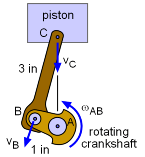Piston Configuration Labels This piston problem can be solved by breaking the problem into two parts. First, find the velocity of point B since the crankshaft angular velocity is known. Next, find the velocity of point C using the velocity of point B. After the velocity of point C is known in terms of the rotation angle θ, the problem could be optimized to find the maximum velocity. Part a) Motion of Bar AB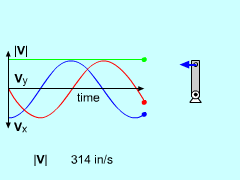Bar AB Motion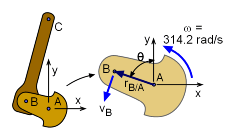Bar AB Diagram Use the relative velocity equation to find the velocity of B. Note that point A does not translate.      vB = vA + vB/A = 0 + ωAB × rB/A Next, the angular velocity, ω = 3,000 rpm, needs to be converted into radians per second.      3000 rpm (1 min/60 s) (2π/1 rev) = 314.2 rad/s The relative distance vector now needs to be defined. Referring to the diagram, we get      rB/A = -|rB/A| sinθi + |rB/A| cosθj             = -1" sinθi + 1" cosθj = -sinθi + cosθj Substituting this into the relative velocity equation above gives the final velocity of point B in terms of the angle θ. The angle θ is still not known but will be determined when the maximum velocity is found.      vB = 314.2k × ( -sinθi + cosθj )           = -314.2 cosθi - 314.2 sinθj in/s Part b) Motion of Bar BC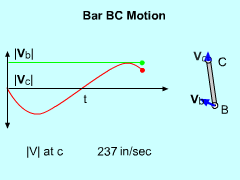Bar BC Motion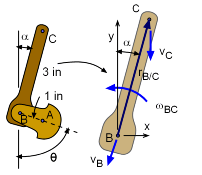Bar BC Diagram Once again, the relative velocity equation is used to determine the velocity of a point on a rotating and translating bar.      vC = vB + vC/B = vB + ωBC × rC/B The velocity of point B is known from previous analysis and the velocity of point C is constrained in the vertical direction. The angular velocity ωBC is not known.      vCj = - 314.2 cosθi - 314.2 sinθj +                         ωBCk × ( 3"sinαi + 3"cosαj ) Completing the cross product and equating the i and j components gives,      i:    0 = -314.2 cosθ - 3ωBC cosα      j:  vC = -314.2 sinθ + 3ωBC sinα The two angles θ and α are actually related (see diagram). The horizontal distance of both arms must equal each other since points A and C always aligned in the vertical direction.      (3 in) sinα = (1 in) sinθ Substituting α = sin-1 (sinθ /3) into the i terms and solving for ωBC gives, gives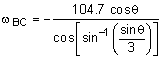This can now be used with the j terms to solve for the velocity of C in terms of the angle θ,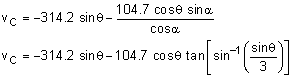Finding Maximum Velocity of Point C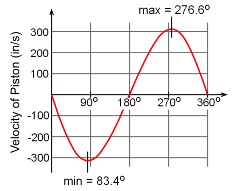Piston Velocity over 360o Motion Up to this point, the velocity vC has been derived for any angle θ. The angle that gives the maximum velocity now needs to be determined. This can be done by taking a derivative with respect to the angle θ and equating it to zero.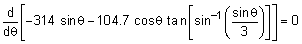Without the help of a symbolic math program such as Mathcad™ or Mathematica™, closed-form derivation becomes nearly impossible. Even then, the result is complex. The final solution, using numerical methods, for the maximum velocity of point C is      θ = 1.455 rad = 83.4o      vmax = +/- 316.3 in/s The velocity curve is plotted for point C in the diagram at the left.

Practice Homework and Test problems now available in the 'Eng Dynamics' mobile app
Includes over 400 problems with complete detailed solutions.
Available now at the Google Play Store and Apple App Store.